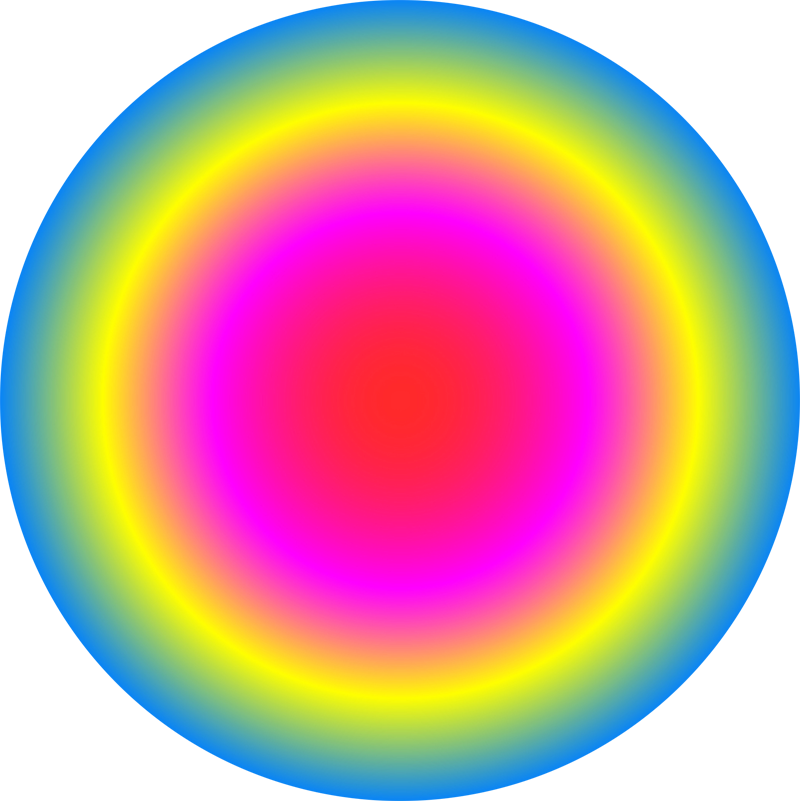# Conor Lawless

## Scientific Computing

data science, dynamic simulation modelling, genomics, interactive visualisation, dashboards, image & video analysis
e: cnr.lwlss@gmail.com
t: @cnrlwlss

## Translating maths into code: writing the tattoo function in Python

In a previous post I discussed a mathematical function which represents a range of different list-comprehensions and how their output can be visualised. Here I’ll describe in detail how we can evaluate this function on a computer, using the Python programming language as an example. Here is the mathematical notation for the tattoo function $t$ again: $$t(\theta, p) = \left\{ \sum_{k=-1}^{n}e^{\frac{2 \pi i k^p}{\theta}} \mid n \in -1,\dots,\theta \right\}$$ What should we expect from mathematical expressions in code? ... Read more

## Mathematical art: tattoos & list-comprehensions

In 2007, as part of a series of collaborations with the visual artist James Johnson-Perkins, I made a video demonstrating the diversity of output possible from a fairly simple mathematical function (see function $t$ below). The video was displayed on large, external, urban screens in Derby, Nottingham and Leicester as part of the ‘Do billboards dream of electric sheep?’ exhibition. The shapes displayed in each of the frames of the video were generated by passing the frame number to a function like $t$: ... Read more

## Image analysis in Python: locating & visualising areas of an image that are in sharp focus

In this post I’ll demonstrate how to locate and highlight the areas of any digital image that are in focus. In microscopy and macro photography, being able to identify image segments that are in focus is important because of the narrow depth-of-field achievable using wide apertures. If you need to construct a high resolution image of a very small subject, you may have to capture a stack of several images (often called a z-stack), each focussed on a different slice of the subject. ... Read more

## Interactive plots for comparing simulated output from simulation models: scientific computing & javascript

In a previous post , I used the interactive plot below to visualise & compare population growth curves simulated from three members of a family of models that share the same set of parameters. The user can adjust parameter values and explore the resulting changes in similarities and differences between simulations from the three models in real time. In this post I will discuss where dynamic visualisation of quantitative analysis is most useful and how the calculations underlying this analysis were carried out in JavaScript. ... Read more

## A family of mathematical models describing population growth

In this post, I will describe a family of three related mathematical models of population dynamics. Since their development, scientists have used these models to simulate the growth of a wide range of different types of populations including the growth of microbial cells, plant growth and the growth of human populations. Each of these three models are written down in the form of ordinary differential equations (ODEs) and are relatively straightforward to simulate from. ... Read more